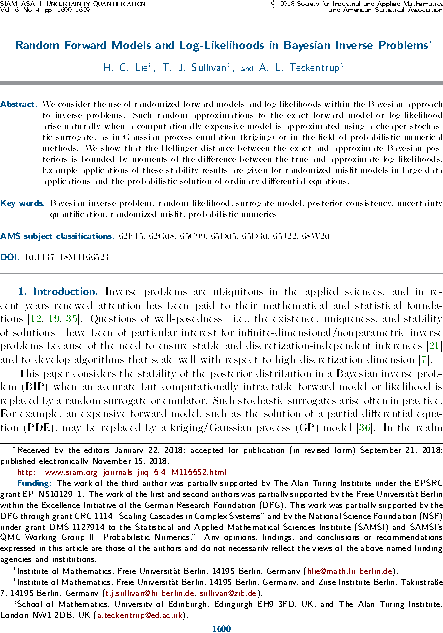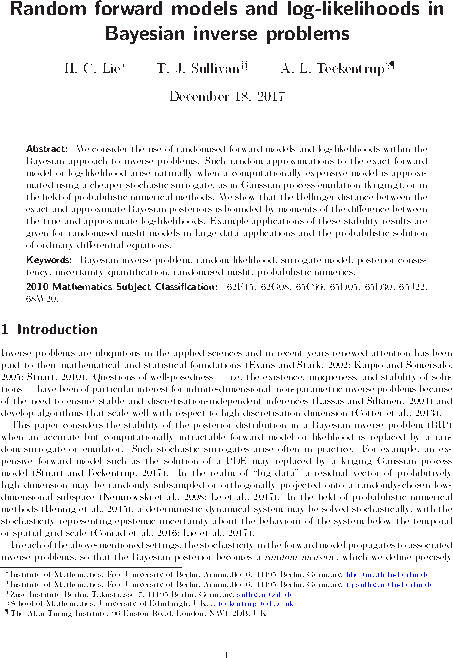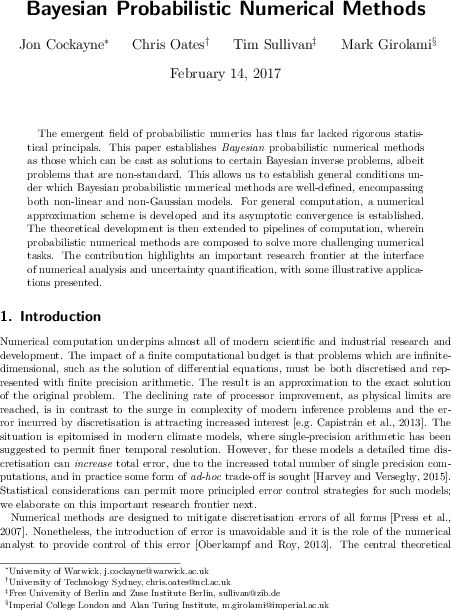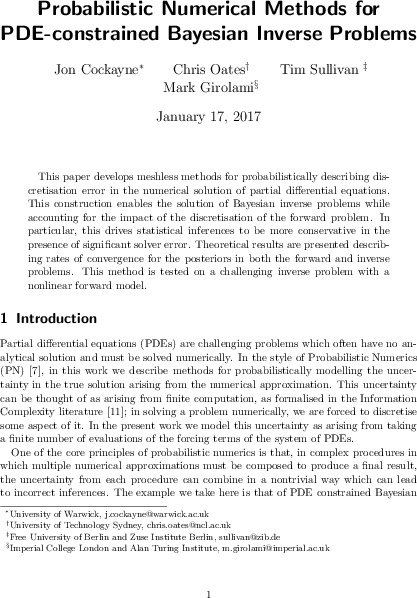# Tim Sullivan

### #bayesian

Clear Search### Random Bayesian inverse problems in JUQ

The article “Random forward models and log-likelihoods in Bayesian inverse problems” by Han Cheng Lie, Aretha Teckentrup, and myself has now appeared in its final form in the SIAM/ASA Journal on Uncertainty Quantification, volume 6, issue 4. This paper considers the effect of approximating the likelihood in a Bayesian inverse problem by a random surrogate, as frequently happens in applications, with the aim of showing that the perturbed posterior distribution is close to the exact one in a suitable sense. This article considers general randomisation models, and thereby expands upon the previous investigations of Stuart and Teckentrup (2017) in the Gaussian setting.

H. C. Lie, T. J. Sullivan, and A. L. Teckentrup. “Random forward models and log-likelihoods in Bayesian inverse problems.” SIAM/ASA Journal on Uncertainty Quantification 6(4):1600–1629, 2018. doi:10.1137/18M1166523

Abstract. We consider the use of randomised forward models and log-likelihoods within the Bayesian approach to inverse problems. Such random approximations to the exact forward model or log-likelihood arise naturally when a computationally expensive model is approximated using a cheaper stochastic surrogate, as in Gaussian process emulation (kriging), or in the field of probabilistic numerical methods. We show that the Hellinger distance between the exact and approximate Bayesian posteriors is bounded by moments of the difference between the true and approximate log-likelihoods. Example applications of these stability results are given for randomised misfit models in large data applications and the probabilistic solution of ordinary differential equations.

Published on Monday 10 December 2018 at 12:00 UTC #publication #bayesian #inverse-problems #juq #prob-num #lie #teckentrup### Preprint: Random Bayesian inverse problems

Han Cheng Lie, Aretha Teckentrup, and I have just uploaded a preprint of our latest article, “Random forward models and log-likelihoods in Bayesian inverse problems”, to the arXiv. This paper considers the effect of approximating the likelihood in a Bayesian inverse problem by a random surrogate, as frequently happens in applications, with the aim of showing that the perturbed posterior distribution is close to the exact one in a suitable sense. This article considers general randomisation models, and thereby expands upon the previous investigations of Stuart and Teckentrup (2017) in the Gaussian setting.

Abstract. We consider the use of randomised forward models and log-likelihoods within the Bayesian approach to inverse problems. Such random approximations to the exact forward model or log-likelihood arise naturally when a computationally expensive model is approximated using a cheaper stochastic surrogate, as in Gaussian process emulation (kriging), or in the field of probabilistic numerical methods. We show that the Hellinger distance between the exact and approximate Bayesian posteriors is bounded by moments of the difference between the true and approximate log-likelihoods. Example applications of these stability results are given for randomised misfit models in large data applications and the probabilistic solution of ordinary differential equations.

Published on Tuesday 19 December 2017 at 08:30 UTC #publication #preprint #bayesian #inverse-problems #lie #teckentrup### Heavy-tailed stable priors in Inverse Problems and Imaging

The final version of “Well-posed Bayesian inverse problems and heavy-tailed stable quasi-Banach space priors” has now been published online in Inverse Problems and Imaging; the print version will be available in October.

T. J. Sullivan. “Well-posed Bayesian inverse problems and heavy-tailed stable quasi-Banach space priors.” Inverse Problems and Imaging 11(5):857–874, 2017. doi:10.3934/ipi.2017040

Published on Wednesday 19 July 2017 at 15:30 UTC #publication #bayesian #inverse-problems #cauchy-distribution #inverse-problems-and-imaging### Preprint: Bayesian probabilistic numerical methods

Jon Cockayne, Chris Oates, Mark Girolami and I have just uploaded a preprint of our latest paper, “Bayesian probabilistic numerical methods” to the arXiv. Following on from our earlier work “Probabilistic meshless methods for partial differential equations and Bayesian inverse problems”, our aim is to provide some rigorous theoretical underpinnings for the emerging field of probabilistic numerics, and in particular to define what it means for such a method to be “Bayesian”, by connecting with the established theories of Bayesian inversion and disintegration of measures.

Abstract. The emergent field of probabilistic numerics has thus far lacked rigorous statistical principals. This paper establishes Bayesian probabilistic numerical methods as those which can be cast as solutions to certain Bayesian inverse problems, albeit problems that are non-standard. This allows us to establish general conditions under which Bayesian probabilistic numerical methods are well-defined, encompassing both non-linear and non-Gaussian models. For general computation, a numerical approximation scheme is developed and its asymptotic convergence is established. The theoretical development is then extended to pipelines of computation, wherein probabilistic numerical methods are composed to solve more challenging numerical tasks. The contribution highlights an important research frontier at the interface of numerical analysis and uncertainty quantification, with some illustrative applications presented.

Published on Tuesday 14 February 2017 at 12:00 UTC #publication #preprint #prob-num #bayesian #cockayne #oates #girolami### Preprint: Probabilistic numerical methods for PDE-constrained Bayesian inverse problems

Jon Cockayne, Chris Oates, Mark Girolami and I have just uploaded a preprint of our latest paper, “Probabilistic numerical methods for PDE-constrained Bayesian inverse problems” to the arXiv. This paper is intended to complement our earlier work “Probabilistic meshless methods for partial differential equations and Bayesian inverse problems” and to give a more concise presentation of the main ideas, aimed at a general audience.

Published on Wednesday 18 January 2017 at 12:00 UTC #publication #preprint #prob-num #bayesian #inverse-problems #cockayne #oates #girolami